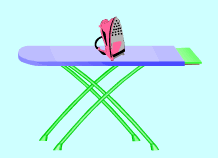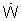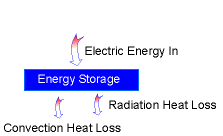Ch 1. Basics Multimedia Engineering Thermodynamics System Temperature& Pressure Heat andWork Energy
 Chapter 1. Basics 2. Pure Substances 3. First Law 4. Energy Analysis 5. Second Law 6. Entropy 7. Exergy Analysis 8. Gas Power Cyc 9. Brayton Cycle 10. Rankine Cycle Appendix Basic Math Units Thermo Tables Search eBooks Dynamics Fluids Math Mechanics Statics Thermodynamics Author(s): Meirong Huang Kurt Gramoll ©Kurt GramollTHERMODYNAMICS - CASE STUDY IntroductionIron and Iron Board Before ironing, an iron needs to be warmed up to a certain temperature. The iron temperature is different for different fabric. The resistance wires are used to generate the energy needed to warm the iron up. Known: The power of the iron is= 100 W and 85% of the heat generated in the resistance wires is transferred to the iron base plate The materials of the base plate is aluminum alloy 2024-76 (ρ = 2770 kg/m3 and cp= 875 J/(kg-oC)) and the base plate thickness δ = 0.5 cm, and the area is A = 0.02 m2 Initially the iron is in equilibrium with the ambient air at Tinitial = 20oC The convection heat transfer coefficient h = 35 W/(m2-oC) The emissivity of the base plate to the ambient ε = 0.6 Questions Determine the minimum time needed for the base plate temperature to reach Tfinal = 140o C. After the temperature reaches 140o C, how much energy is needed to keep the base at 140o C? ApproachEnergy Balance Diagram The heat needed to increase the base plate's temperature from Tinital to Tfinal is: Q = cp m (Tfinal - Tinitial) where m = mass of the base cp = specific heat The energy balance of the base plate (Energy balance will be introduced in the following section): Energy in: 85% of the heat generated by the wires. Energy out: convection, radiation heat loss to the ambient air. Energy storage: the energy in the base to increase the base's temperature. Ein - Eout = Estorage